﻿ Kinematic Analysis of Planar Snake-like Robot Mechanism Using of Matrices Formulation

Kinematic Analysis of Planar Snake-like Robot Mechanism Using of Matrices Formulation

Erik Prada, Alexander Gmiterko, Tomáš Lipták, Ľubica Miková, František MendaOPEN ACCESSPEER-REVIEWED

Kinematic Analysis of Planar Snake-like Robot Mechanism Using of Matrices Formulation

Erik Prada1,, Alexander Gmiterko1, Tomáš Lipták1, Ľubica Miková1, František Menda1

1Technical University of Košice, Department of Applied Mechanics and Mechatronics, Košice, Slovakia

Abstract

The article deals with the kinematics of a three section snake-like robot. In the introductory part of the article, the main advantages and application possibilities of a snake-like movement in practice are mentioned. In the next chapter, the mathematical model of a direct kinematics of a three link snake-like robot was created. It also includes the fourth, variable “virtual” link. Mathematical model was numerically confirmed in the conclusion. Homogeneous transformational matrices were used for the creation of mathematical model. Subsequently, positional vectors of centers of mass all links were expressed. For the derivation of velocity vectors centers of mass, the numerical methods with the application of differential operators were used. In the conclusion of the work, the graphical presentation of the centers of mass positions is illustrated. They were realized in the Matlab 2012 programming environment.

At a glance: Figures

12
Prev Next

• Prada, Erik, et al. "Kinematic Analysis of Planar Snake-like Robot Mechanism Using of Matrices Formulation." American Journal of Mechanical Engineering 1.7 (2013): 447-450.
• Prada, E. , Gmiterko, A. , Lipták, T. , Miková, Ľ. , & Menda, F. (2013). Kinematic Analysis of Planar Snake-like Robot Mechanism Using of Matrices Formulation. American Journal of Mechanical Engineering, 1(7), 447-450.
• Prada, Erik, Alexander Gmiterko, Tomáš Lipták, Ľubica Miková, and František Menda. "Kinematic Analysis of Planar Snake-like Robot Mechanism Using of Matrices Formulation." American Journal of Mechanical Engineering 1, no. 7 (2013): 447-450.

 Import into BibTeX Import into EndNote Import into RefMan Import into RefWorks

1. Introduction

The reason why biologists and engineers are engaged in the solving of the snake movements is that these movements are interesting in case of its use in various situations. Mechatronic systems imitating the snake’s movement can be used for activities such as research of dimensionally confined space, search for people after earthquakes, pipe inspection systems, inspection of spaces, which are harmful to human health, use for spy purposes, use for the study of other animals and finally, for the research of live snakes behavior in their natural environment [1, 3, 4].

Currently, there are solutions of mechatronic snakes of different uses from surgery to military industry as seen on the Figure 1.

2. Direct Kinematics Solution of a Snake-like Robot

We have applied the knowledge of basic mathematical structures, more specifically matrix methods, in addressing the problems of direct kinematics of a snake-like robot. Direct kinematics may be defined in such a way that before the realization of a calculation, we know the dimensions of a mechanism and the joint coordinates of the whole chain with n segments which are represented by the transposed vector q [2, 4].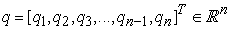(1)

The aim of the direct kinematics is to find the coordinates of the vector sought, whether it is a position vector of a specified point x, velocity vector of a given point v, or the acceleration vector a. Displacement, rotation or a combination of these movements is characterized by homogenous coordinates qi. All mutual joints between the links in the chain may be realized by rotary, translational or the combination of both of these joints. Depending on the joints and segments used, there is a direct link with the number of homogeneous coordinates describing the configuration options of a chain. Translational and rotational joints in the plane have one degree of freedom of movement.

The general algorithm of the n segment kinematics of a kinematical chain calculation consists of the following steps [1, 2]:

Ÿ  Creation and definition of vector equations for determination of the position, velocity and acceleration vectors of a point sought,

Ÿ  Creation of a transformation matrix between the n system and the global frame with the use of homogeneous transformation equations between the two successive coordinate systems, which have the following form for n segments [1, 2]: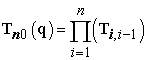(2)

Ÿ  By instituting the standard transformation matrix into the Equation (2), we obtain the vector equation for determining the position vector of a last segment point sought [1, 2]: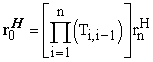(3)

Ÿ  To determine the velocity vector of an H point with respect to the beginning of a coordinate system, it is necessary to conduct the derivation of a transformation matrix according to time and subsequently install it into equation [1, 2]: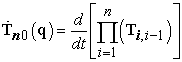(4)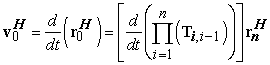(5)

Ÿ  It is necessary to create a second derivation of a transformation matrix according to time to determine the acceleration vector of H point analogously [1, 2]: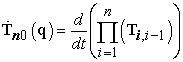(6)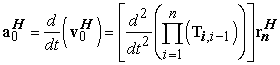(7)

3. Solution of a Case with Rotary Joints by Numerical Matrix Method

We use the Equation (3) to determine the position vectors of the segments linked by center of rotary joints. The form of the position vectors in centers of mass a second and first head segment is as follows: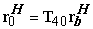(8)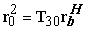(9)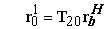(10)

We have determined the transformation matrices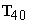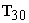,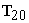by the correct multiplication of the transformation matrices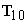,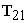,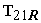,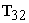,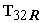,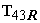, whose form is due to a kinematical chain in with rotary joint as follows :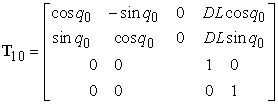(11)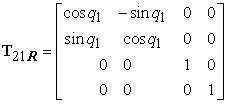(12)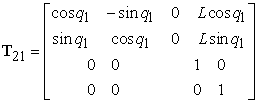(13)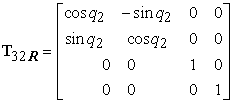(14)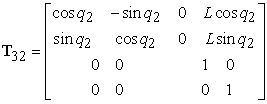(15)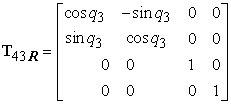(16)

Vector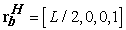. Transformation matrixserves to rotate the coordinate system from the global origin about the angle corresponding to coordinate q0 and at the same time for the axial displacement in the direction of the section’s axis about the value DL. However, this is a simulation of a virtual section connected with the global frame of the global coordinate system. Transmission of a coordinate system thus reaches the marginal position (position of a tail) of a segment 1. The matrixrepresents a transformation of a coordinate system from the beginning of a first segment of a three link snake-like robot to a place of rotary joints between the first and the second segment. The matrix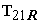serves only to rotate the coordinate system in a tail part of the snake and the matrix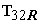serves to rotate the coordinate system in a joint between the tail and the middle segment. The transformation matrixdescribes the transformation of a coordinate system from the space of a rotary joint between the first and the second segment to a space of the second joint between the second and the third segment together with a rotation. The last matrixdescribes the rotation of a coordinate system between the middle and the head segment. The constant L represents the distance between the joints, which corresponds to the length of one segment of a snake in a simplified form [1, 5].

Rotation around the first, the second and the third segment is characterized by the coordinates q1, q2 and q3 .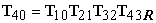(17)

We got the transformation matrixby the multiplication of all the previous matrices in a certain order. By installing the transformation matrixinto the Equation (8) we got the final form of the position vector of a head center of mass of a snake-like robot Equation (18). Vector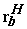is the position vector of a head segment center relative to the last local coordinate system placed in the rotary joint between the head and the second segment .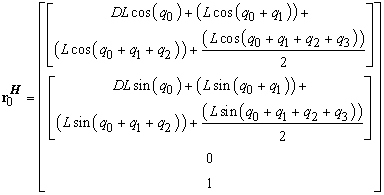(18)

Since we have determined the position vectors of the centers of mass of kinematical segments of a chain, we can further determine the velocity vectors of individual segments. For the determination, we will use an equation for the calculation of a velocity matrix. For the determination of the velocity vectors, we will derive the relevant transformation matrix. Derivation will be carried out by the numerical method with the use of differential operators of the transformation matrices. Velocity vectors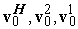of a snake-like robot segments will be calculated with the use of the equations: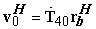(19)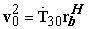(20)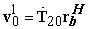(21)

Vectors are equal to the conjunction of the transformation matrices derivations and the terminal position vectorin the final coordinate system, in which the last transformation took place. Derivation of a transformation matrix of a head segment has the following form:(22)

Derivations of transformation matrices needed to calculate the total derivation will be determined as a multiplication of a transformation matrix of the respective transformation and the velocity matrix.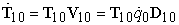(23)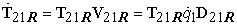(24)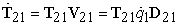(25)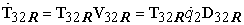(26)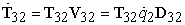(27)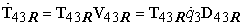(28)

Matrices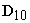from to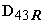are differential operators needed to calculate the velocity matrices from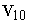to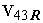. The variables from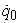to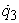in the equations of the calculation of velocity matrices are derivations of general coordinates and correspond to the velocity angles of virtual rotary joints and real rotary joints between the sections.

In case of real rotary joints, the angle regards velocity of the positioning of rotary actuators. By multiplying the transformation matrices gradually according to the indexes, we will get the derivation of the final transformation matrix of the head segment center of mass [1, 5].

4. Computational Verification of a Kinematics of Three Link Snake-like Robot with Rotary Joints

We used the derived equations of position vectors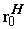and velocity vectors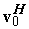for the calculation of the head segment of a three section snake-like robot with the rotary joints. The calculation consists in the institution of numerical values into the resultant relations of position vectors. We used the programming environment MATLAB/Simulink 2012 for the calculation [1, 2].

Ÿ  DL – length of the virtual segment between the solid frame SS and the end of the tail section

Ÿ  L – length of the real segment of the snake-like robot

Ÿ  q0 –general coordinate of a rotation of a virtual joint, which connects the virtual segment with the global origin

Ÿ  q1 – general coordinate of the rotation of a joint between the virtual and tail segment

Ÿ  q2 – general coordinate of the rotation of a joint between the first and the second segment

Ÿ  q3 – general coordinate of the rotation of a joint between the second and the head segment

Table 1. Table of the test configurations of the input values of the calculationDownload asPowerPoint Slide

Veiw figureView current table in a new window

We chose the command to create the vector in the range (from 0 to 100) for DL. We wanted to achieve the constant enlarging of the distance of other sections from the origin of the coordinate system and simulate some form of the forward progress of the whole chain by that. The length of a real segment during the whole calculation is constant and equal to L=120 mm. General coordinates of the joint rotation were changed throughout the course of the calculation according to the configurations shown in the Table. 1. Rotation values were featured in radians and we used the maximum possible rotation that the construction permits, which equals .

Simulation outputs were exported to the data type of the field from which we subsequently generated the required dependence graphs. For each of the configuration settings of the input values, we have created the output dependence graphs Figure 1, Figure 2 and Figure 3.

The position graphs depict the dependence of the calculated values of axis-y of the position vector on the values of axis-x of a position vector.

Figure 4. The CG of the head, middle and tail segment in the XY plane in configuration 3 

5. Conclusion

Results of kinematical analysis conducted in Chapter 4 give us the basic overview about the mutual position of individual centers of mass of three link robot moving in the plane. The analysis was conducted in three different configuration settings parameters of individual joint coordinates. Due to the fact that the linear vector with a finite number of points was used to change the values of these coordinates, unique linear combinations for each of the configurations were created. Along with these combinations, there was a gradual increase of the size of a virtual segment taking place, which does not correspond to reality completely.

Acknowledgement

The authors would like to thank to Slovak Grant Agency – project VEGA 1/1205/12 “Numerical modeling of mechatronic systems”. This contribution is also result of the project VEGA MŠ SR for the support of this work under Project No. 1/0937/12. This contribution is also result of the project APVV-0091-11 “Using of methods of experimental and numerical modeling for increasing of competitiveness and innovation of mechanical and mechatronical systems”.

References

  Prada, E., Kinematic analysis of planar snake-like robot, little doctoral exam thesis. Faculty of mechanical Engineering, Technical university of Kosice, Kosice, 2012.In article  Grepl, R., Kinematika a dynamika mechatronických systému. Brno 2007.In article PubMed  Gray, J., “The mechanism of locomotion in snakes”, The Journal of Experimental Biology, Vol. 23, No. 2, pp. 101 - 120, 1946.In article PubMed  Transeth, A.A., Modelling and Control of Snake Robots, Doctoral Thesis, Norwegian University of Science and Technology, 2007.In article  Chirikjian, G., Burdick, J., “Kinematics of hyper-redundant robot locomotion with application to grasping”, In proceeding of the IEEE International conference on robotics and automation, pp. 720 -725, California, 1991.In article